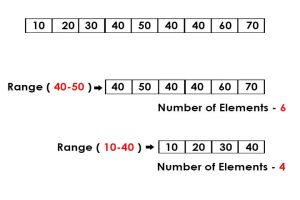# Python | Numbers in a list within a given range

Given a list, print the number of numbers in the given range.Examples:

```Input : [10, 20, 30, 40, 50, 40, 40, 60, 70] range: 40-80
Output : 6

Input : [10, 20, 30, 40, 50, 40, 40, 60, 70] range: 10-40
Output : 4
```

## Recommended: Please try your approach on {IDE} first, before moving on to the solution.

Multiple Line Approach:

Traverse in the list and check for every number. If the number lies in the specified range, then increase the counter. At the end of traversal, the value of the counter will be the answer for the number of numbers in specified range.

Below is the Python implementation of the above approach

 `# Python program to count the  ` `# number of numbers in a given range ` `# using traversal and mutliple line code ` ` `  `def` `count(list1, l, r): ` `    ``c ``=` `0`  `    ``# traverse in the list1 ` `    ``for` `x ``in` `list1: ` `        ``# condition check ` `        ``if` `x>``=` `l ``and` `x<``=` `r: ` `            ``c``+``=` `1`  `    ``return` `c ` `     `  `# driver code ` `list1 ``=` `[``10``, ``20``, ``30``, ``40``, ``50``, ``40``, ``40``, ``60``, ``70``] ` `l ``=` `40` `r ``=` `80`  `print` `count(list1, l, r) `

Output:

```6
```

Single Line Approach:

We can write a single line for traversal and checking condition together:

`x for x in list1 if l <= x <= r`

The return value(true) of the condition check is stored in a list, and at the end the length of the list returns the answer.

Below is the Python implementation of the above approach

 `# Python program to count the  ` `# number of numbers in a given range ` ` `  `def` `count(list1, l, r): ` `     `  `    ``# x for x in list1 is same as traversal in the list ` `    ``# the if condition checks for the number of numbers in the range  ` `    ``# l to r  ` `    ``# the return is stored in a list ` `    ``# whose length is the answer ` `    ``return` `len``(``list``(x ``for` `x ``in` `list1 ``if` `l <``=` `x <``=` `r)) ` ` `  `# driver code ` `list1 ``=` `[``10``, ``20``, ``30``, ``40``, ``50``, ``40``, ``40``, ``60``, ``70``] ` `l ``=` `40` `r ``=` `80`  `print` `count(list1, l, r) `

Output:

```6
```

My Personal Notes arrow_drop_upCheck out this Author's contributed articles.

If you like GeeksforGeeks and would like to contribute, you can also write an article using contribute.geeksforgeeks.org or mail your article to contribute@geeksforgeeks.org. See your article appearing on the GeeksforGeeks main page and help other Geeks.

Please Improve this article if you find anything incorrect by clicking on the "Improve Article" button below.

Article Tags :
Practice Tags :

2

Please write to us at contribute@geeksforgeeks.org to report any issue with the above content.